# 5vCircuitPowerMeter

Make a power meter for 5v circuits using material from the Arduino Starter Kit

IntermediateFull instructions provided2,451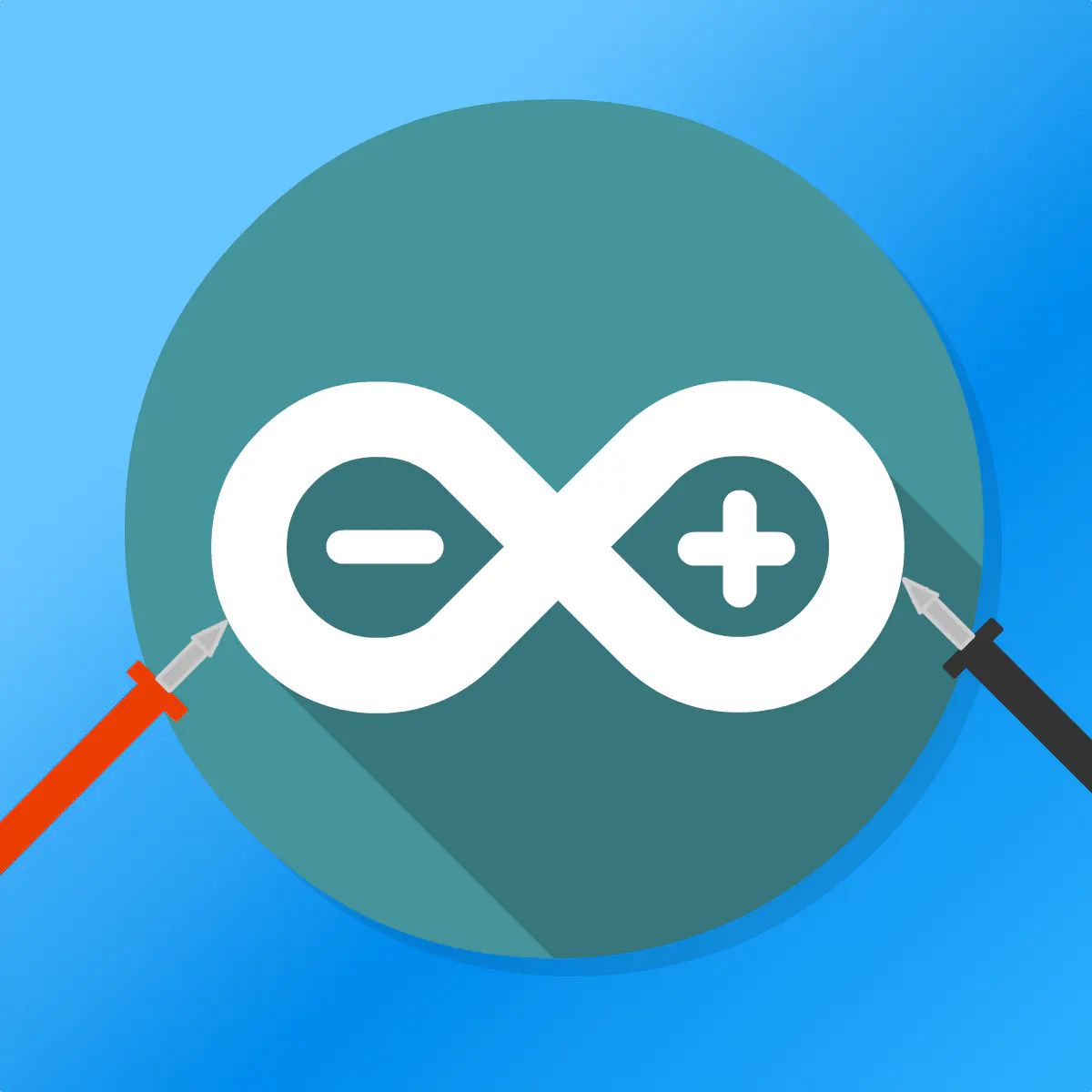## Things used in this project

### Hardware componentsArduino UNO
×1Jumper wires (generic)
×18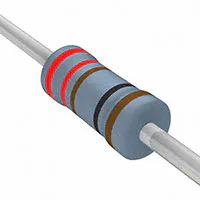Resistor 221 ohm
×1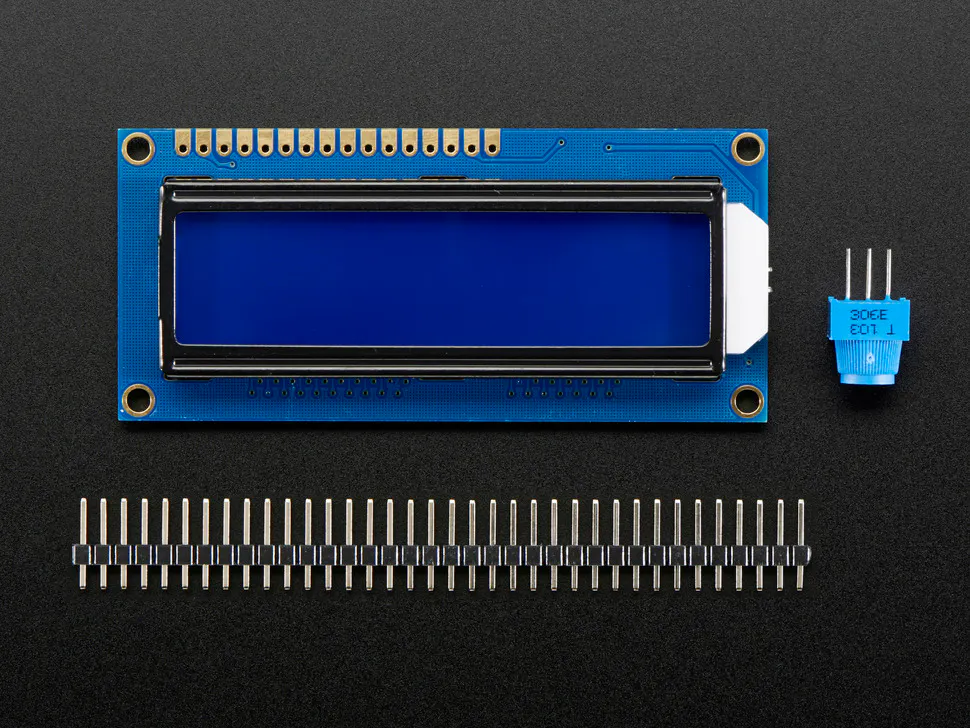Adafruit Standard LCD - 16x2 White on Blue
×1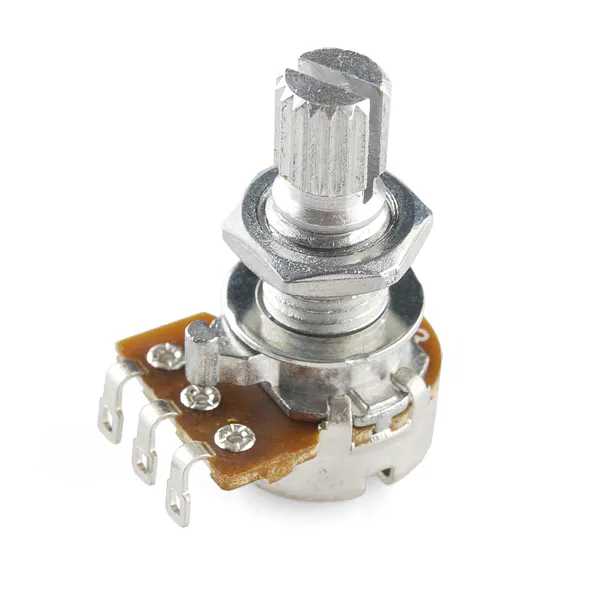Rotary potentiometer (generic)
×1

### Software apps and online servicesArduino IDE

## Schematics

### Main Project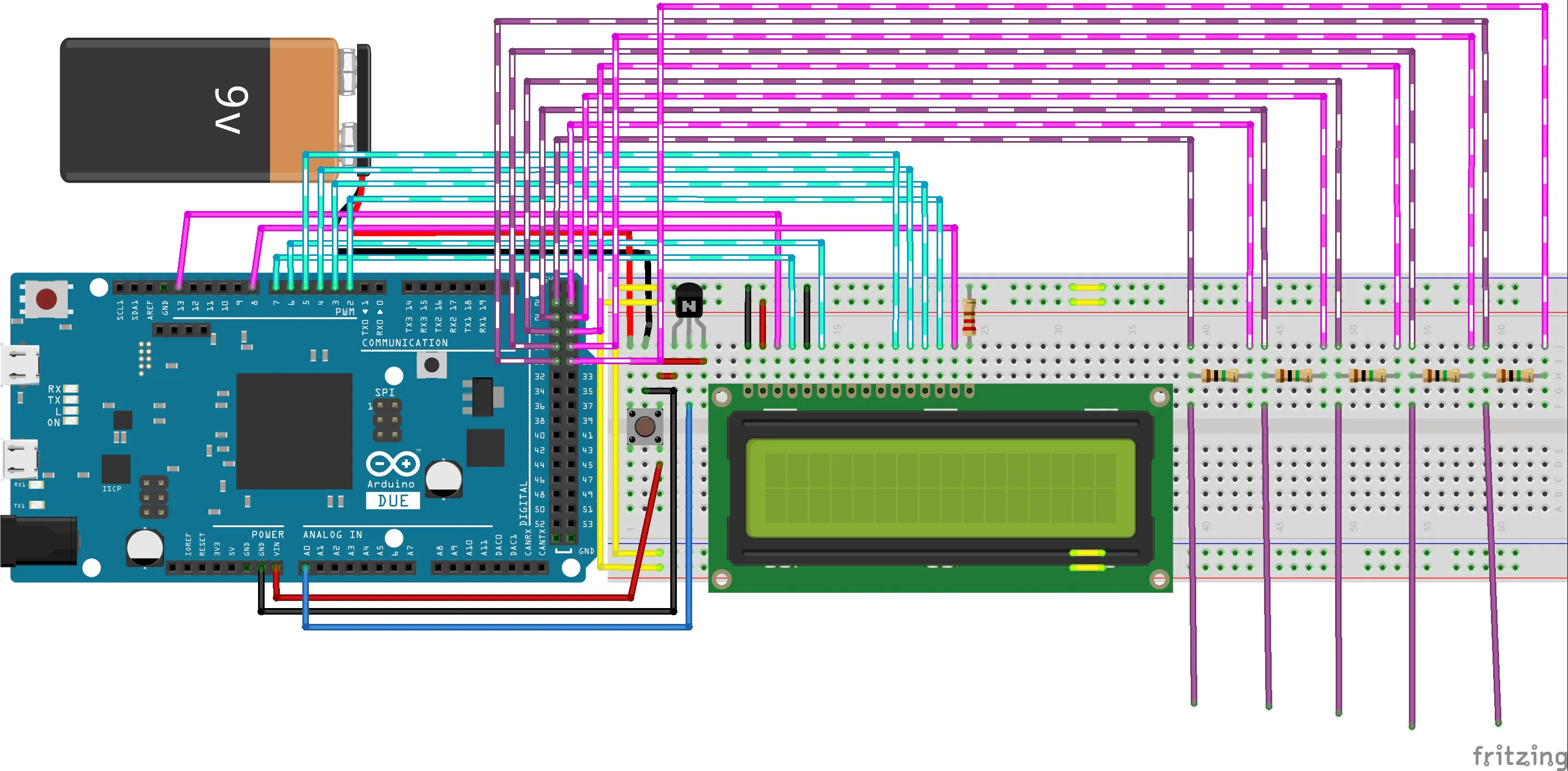### Main Project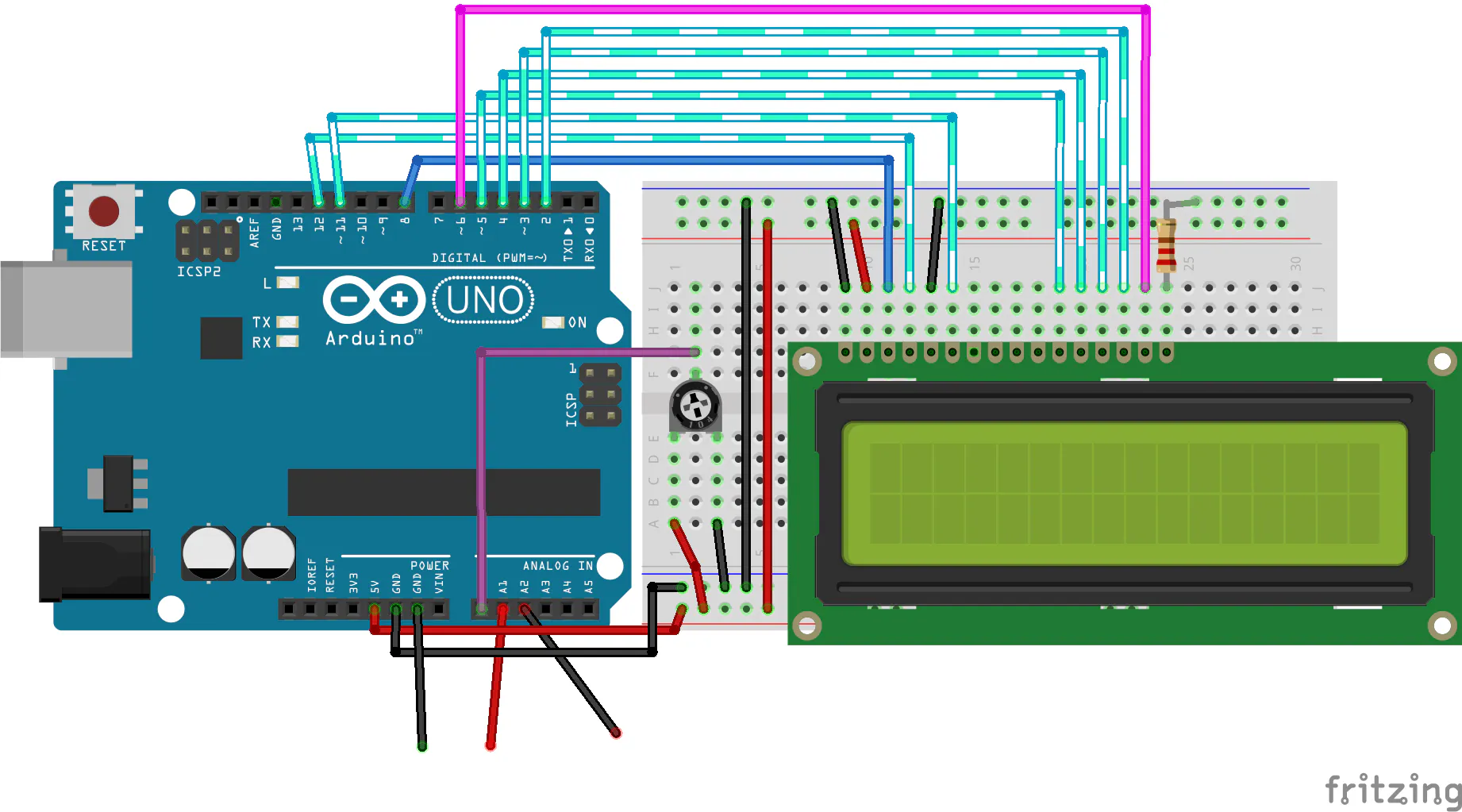### LCD Setup

How to setup a 16x2 LCD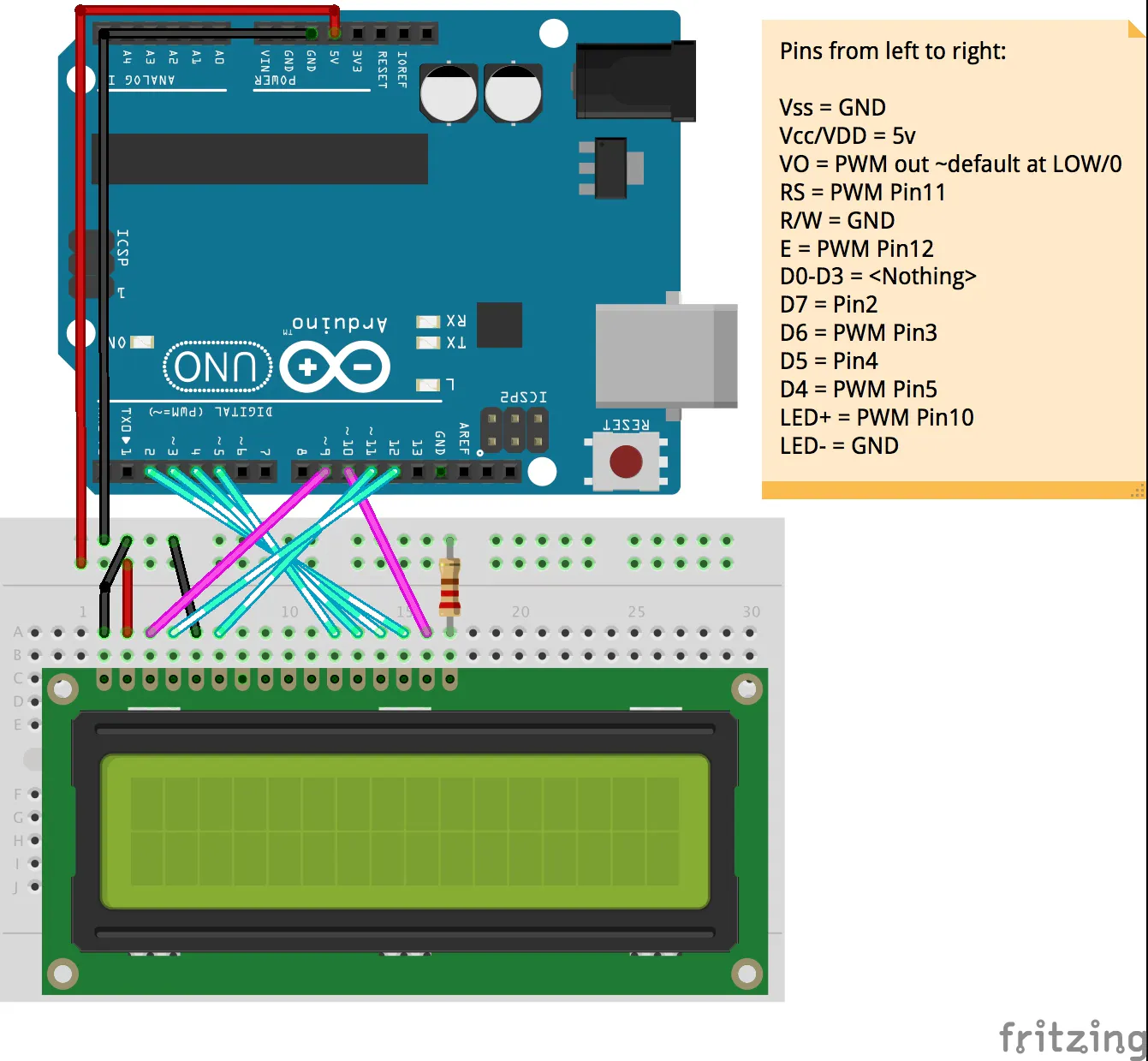## Code

### PowerMeter

Arduino
Main code
```#include <LiquidCrystal.h>

//Include^
//Init
LiquidCrystal lcd(12, 11, 5, 4, 3, 2);

//Global Var
int pot = 0;
int index = 0;

const int out = A1;
const int in = A2;
const int mm = A0;
const int li = 6;
void setup() {//~~~~~~~~~~~~~~~~~~~~~~~~~~~~~~~~~~~~~~~~~~~~~
lcd.begin(16, 2);
// put your setup code here, to run once:
pinMode(li, OUTPUT);
pinMode(out, OUTPUT);
pinMode(in, INPUT);
pinMode(mm, INPUT);
pinMode(8, OUTPUT);
off(8);
on(li);
lcd.print("   PowerMeter");
delay(1000);
lcd.clear();

}//~~~~~~~~~~~~~~~~~~~~~~~~~~~~~~~~~~~~~~~~~~~~~

void loop() {//~~~~~~~~~~~~~~~~~~~~~~~~~~~~~~~~~~~~~~~~~~~~~
// put your main code here, to run repeatedly:
index = map(pot, 0, 1023, 1, 5);

if (index == 1) {
off(li);
on(8);
}
if (index == 2) {
lcd.clear();
on(li);
off(8);
lcd.print(" DC Volt Meter");
while (true) {
float vv = analogRead(in) * (5.0 / 1023.0);
lcd.setCursor(0, 1);
lcd.print(vv);

index = map(pot, 0, 1023, 1, 5);
if (index != 2)
break;
}
}
if (index == 3) {
lcd.clear();
on(li);
off(8);
lcd.print(" Analog R/W Val");
while (true) {
int aW = map(aR, 0, 1023, 0, 255);
lcd.setCursor(0, 1);
lcd.print("R:" + String(aR) + "     W:" + String(aW)+"    ");

index = map(pot, 0, 1023, 1, 5);
if (index != 3)
break;
}
}
if (index == 4) {
lcd.clear();
on(li);
off(8);
lcd.print("      Ohms");
while (true) {
float vv = analogRead(in) * (5.0 / 1023.0);
float rr = vv / 20.0;
lcd.setCursor(0, 1);
lcd.print(rr);

index = map(pot, 0, 1023, 1, 5);
if (index != 4)
break;
}
}
if (index == 5) {
lcd.clear();
on(li);
off(8);
lcd.print("  Conductivity");
while (true) {
on(out);
lcd.setCursor(0, 1);
lcd.print("Connect         ");
else
lcd.print("Disconnect      ");

index = map(pot, 0, 1023, 1, 5);
if (index != 5)
break;
}
}
}//~~~~~~~~~~~~~~~~~~~~~~~~~~~~~~~~~~~~~~~~~~~~~

//Functions
//New~~~~~~~~~~~~~~~~

//Back~~~~~~~~~~~~~~~~~~
void on(int pin) {
digitalWrite(pin, HIGH);
}
void off(int pin) {
digitalWrite(pin, LOW);
}
```

## Credits

### MicroBob

8 projects • 88 followers
Maker, developer, 3D content creator